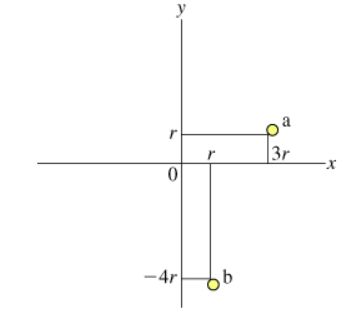# Problem: Part AWhat is the moment of inertia Ia of particle a?a.  mr2b. 9mr2c. 10mr2d. undefined: an axis of rotation has not been specified.Part BFind the moment of inertia Ia,x of particle a with respect to the x axis (that is, if the x axis is the axis of rotation). Express your answer in terms of m and r.Part CFind the moment of inertia Ia,y of particle a with respect to the y axis. Express your answer in terms of m and r.

###### FREE Expert Solution

The moment of inertia is expressed as:

$\overline{){\mathbf{I}}{\mathbf{=}}{\mathbit{M}}{{\mathbit{R}}}^{{\mathbf{2}}}}$, where M is the mass of the object and R is the perpendicular distance.

Part A.

The moment of inertia, mr2 = m(3r)2 = m9r2.

The moment of inertia depends on the axis of rotation.

90% (460 ratings)###### Problem DetailsPart A

What is the moment of inertia Ia of particle a?
a.  mr2
b. 9mr2
c. 10mr2
d. undefined: an axis of rotation has not been specified.

Part B

Find the moment of inertia Ia,x of particle a with respect to the x axis (that is, if the x axis is the axis of rotation).